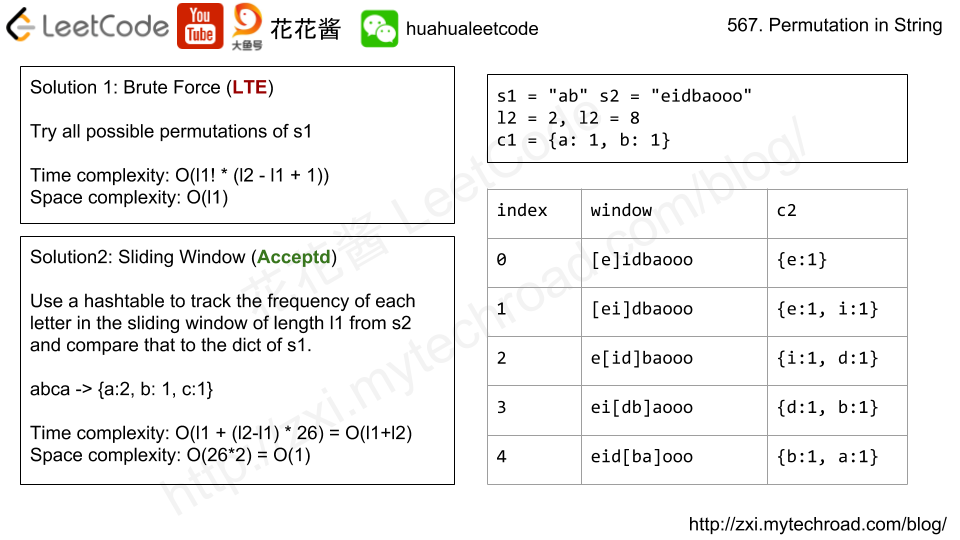# Posts tagged as “substring”

Given a string text, we are allowed to swap two of the characters in the string. Find the length of the longest substring with repeated characters.

Example 1:

Input: text = "ababa"
Output: 3
Explanation: We can swap the first 'b' with the last 'a', or the last 'b' with the first 'a'. Then, the longest repeated character substring is "aaa", which its length is 3.


Example 2:

Input: text = "aaabaaa"
Output: 6
Explanation: Swap 'b' with the last 'a' (or the first 'a'), and we get longest repeated character substring "aaaaaa", which its length is 6.


Example 3:

Input: text = "aaabbaaa"
Output: 4


Example 4:

Input: text = "aaaaa"
Output: 5
Explanation: No need to swap, longest repeated character substring is "aaaaa", length is 5.


Example 5:

Input: text = "abcdef"
Output: 1


Constraints:

• 1 <= text.length <= 20000
• text consist of lowercase English characters only.

## Solution: HashTable

Pre-processing

1. Compute the longest repeated substring starts and ends with text[i].
2. Count the frequency of each letter.

Main

1. Loop through each letter
2. Check the left and right letter
• if they are the same, len = left + right
• e.g1. “aa c aaa [b] aaaa” => len = 3 + 4 = 7
• if they are not the same, len = max(left, right)
• e.g2. “aa [b] ccc d c” => len = max(2, 3) = 3
3. If the letter occurs more than len times, we can always find an extra one and swap it with the current letter => ++len
• e.g.1, count[“a”] = 9 > 7, len = 7 + 1 = 8
• e.g.2, count[“c”] = 4 > 3, len = 3 + 1 = 4

Time complexity: O(n)
Space complexity: O(n)

## C++

You are given a string, s, and a list of words, words, that are all of the same length. Find all starting indices of substring(s) in sthat is a concatenation of each word in words exactly once and without any intervening characters.

Example 1:

Input:
s = "barfoothefoobarman",
words = ["foo","bar"]
Output: [0,9]
Explanation: Substrings starting at index 0 and 9 are "barfoor" and "foobar" respectively.
The output order does not matter, returning [9,0] is fine too.


Example 2:

Input:
s = "wordgoodgoodgoodbestword",
words = ["word","good","best","word"]
Output: []

## Solution1: HashTable + Brute Force

Time complexity: O((|S| – |W|*l) * |W|*l))
Space complexity: O(|W|*l)

# Problem

https://leetcode.com/problems/reorder-log-files/description/

You have an array of logs.  Each log is a space delimited string of words.

For each log, the first word in each log is an alphanumeric identifier.  Then, either:

• Each word after the identifier will consist only of lowercase letters, or;
• Each word after the identifier will consist only of digits.

We will call these two varieties of logs letter-logs and digit-logs.  It is guaranteed that each log has at least one word after its identifier.

Reorder the logs so that all of the letter-logs come before any digit-log.  The letter-logs are ordered lexicographically ignoring identifier, with the identifier used in case of ties.  The digit-logs should be put in their original order.

Return the final order of the logs.

Example 1:

Input: ["a1 9 2 3 1","g1 act car","zo4 4 7","ab1 off key dog","a8 act zoo"]
Output: ["g1 act car","a8 act zoo","ab1 off key dog","a1 9 2 3 1","zo4 4 7"]


Note:

1. 0 <= logs.length <= 100
2. 3 <= logs[i].length <= 100
3. logs[i] is guaranteed to have an identifier, and a word after the identifier.

# Solution: Partition + Sort

1. partition the array such that all digit logs are after all letter logs
2. sort the letter logs part based on the log content

Time complexity: O(n + aloga)

Space complexity: O(n)

# Problem

Implement strStr().

Return the index of the first occurrence of needle in haystack, or -1 if needle is not part of haystack.

Example 1:

Input: haystack = "hello", needle = "ll"
Output: 2


Example 2:

Input: haystack = "aaaaa", needle = "bba"
Output: -1


Clarification:

What should we return when needle is an empty string? This is a great question to ask during an interview.

For the purpose of this problem, we will return 0 when needle is an empty string. This is consistent to C’s strstr() and Java’s indexOf().

# Solution 1: Brute Force

Time complexity: O(mn)

Space complexity: O(1)

# Problem

https://leetcode.com/problems/permutation-in-string/description/

Given two strings s1 and s2, write a function to return true if s2 contains the permutation of s1. In other words, one of the first string’s permutations is the substring of the second string.

Example 1:

Input:s1 = "ab" s2 = "eidbaooo"
Output:True
Explanation: s2 contains one permutation of s1 ("ba").


Example 2:

Input:s1= "ab" s2 = "eidboaoo"
Output: False


Note:

1. The input strings only contain lower case letters.
2. The length of both given strings is in range [1, 10,000].# Solution: Sliding Window

Time Complexity: O(l1 + l2 * 26) = O(l1 + l2)

Space Complexity: O(26 * 2) = O(1)

C++

# Related Problems

Mission News Theme by Compete Themes.